Two masses M and m are connected by a weightless string. They are pulled by a force F on a frictionless horizontal surface. The acceleration of mass m is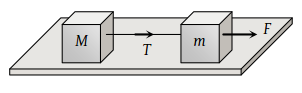(1) $\frac{F}{m}$

(2) $\frac{F-T}{m}$

(3) $\frac{F+T}{m}$

(4) $\frac{F}{M}$

Concept Questions :-

Types of forces
High Yielding Test Series + Question Bank - NEET 2020

Difficulty Level:

A uniform rope of length l lies on a table. If the coefficient of friction is μ, then the maximum length l1 of the part of this rope which can overhang from the edge of the table without sliding down is

(1) $\frac{l}{\mu }$

(2) $\frac{l}{\mu +l}$

(3) $\frac{\mu l}{1+\mu }$

(4) $\frac{\mu l}{\mu -1}$

Concept Questions :-

Friction
High Yielding Test Series + Question Bank - NEET 2020

Difficulty Level:

A heavy uniform chain lies on a horizontal table-top. If the coefficient of friction between the chain and table surface is 0.25, then the maximum fraction of length of the chain, that can hang over one edge of the table is

(1) 20%

(2) 25%

(3) 35%

(4) 15%

Concept Questions :-

Friction
High Yielding Test Series + Question Bank - NEET 2020

Difficulty Level:

The blocks A and B are arranged as shown in the figure. The pulley is frictionless. The mass of A is 10 kg. The coefficient of friction of A with the horizontal surface is 0.20. The minimum mass of B to start the motion will be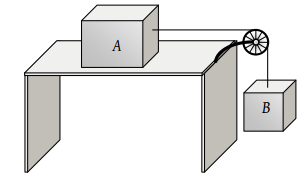(1) 2 kg

(2) 0.2 kg

(3) 5 kg

(4) 10 kg

Concept Questions :-

Types of forces
High Yielding Test Series + Question Bank - NEET 2020

Difficulty Level:

A uniform chain of length L hangs partly from a table which is kept in equilibrium by friction. The maximum length that can withstand without slipping is l, then coefficient of friction between the table and the chain is

(1) $\frac{l}{L}$

(2) $\frac{l}{L+l}$

(3) $\frac{l}{L-l}$

(4) $\frac{L}{L+l}$

Concept Questions :-

Friction
High Yielding Test Series + Question Bank - NEET 2020

Difficulty Level:

When two surfaces are coated with a lubricant, then they

(1) Stick to each other

(2) Slide upon each other

(3) Roll upon each other

(4) None of these

Concept Questions :-

Friction
High Yielding Test Series + Question Bank - NEET 2020

Difficulty Level:

A 20 kg block is initially at rest on a rough horizontal surface. A horizontal force of 75 N is required to set the block in motion. After it is in motion, a horizontal force of 60 N is required to keep the block moving with constant speed. The coefficient of static friction is

(1) 0.38

(2) 0.44

(3) 0.52

(4) 0.60

Concept Questions :-

Friction
High Yielding Test Series + Question Bank - NEET 2020

Difficulty Level:

A block A with mass 100 kg is resting on another block B of mass 200 kg. As shown in figure a horizontal rope tied to a wall holds it. The coefficient of friction between A and B is 0.2 while coefficient of friction between B and the ground is 0.3. The minimum required force F to start moving B will be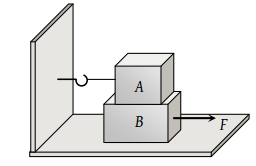(1) 900 N

(2) 100 N

(3) 1100 N

(4) 1200 N

Concept Questions :-

Friction
High Yielding Test Series + Question Bank - NEET 2020

Difficulty Level:

A horizontal force of 10 N is necessary to just hold a block stationary against a wall. The coefficient of friction between the block and the wall is 0.2. The weight of the block is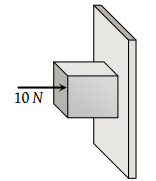(1) 2 N

(2) 20 N

(3) 50 N

(4) 100 N

Concept Questions :-

Types of forces
High Yielding Test Series + Question Bank - NEET 2020

Difficulty Level:

The coefficient of static friction, μs, between block A of mass 2 kg and the table as shown in the figure is 0.2. What would be the maximum mass value of block B so that the two blocks do not move? The string and the pulley are assumed to be smooth and massless. (g = 10 m/s2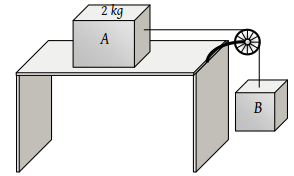(1) 2.0 kg

(2) 4.0 kg

(3) 0.2 kg

(4) 0.4 kg

Concept Questions :-

String constraint